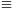Mathematical and Physical Journal
for High Schools
Issued by the MATFUND Foundation
 Already signed up? New to KöMaL?

#Problem A. 462. (October 2008)

A. 462. Let p be an odd prime and let 1<a<p be an integer. Prove that p dividesif and only if there exists an odd positive integer k for which ak is congruent to 1 modulo p.

(5 pont)

Deadline expired on December 15, 2008.

Solution. Let r be the order of the residue a modulo p,

(a) If r is odd, then(b) If r is even, r=2s, then as-1 (mod p).

Let. We will show that ST is not divisible by p.If r|2m, then a2m1 (mod p), and. In all other casesIf m/s is an even integer then m is even, -am-1 mod p), and (-am)-m((-1)2)m/21 (mod p).

If m/s is an odd integer then am(as)m/s-1 (mod p) és (-am)-m1m1 (mod p).

As we have seen, we obtain nonzero residues only when s divides m, and this residue is -1in all such cases. Therefore,Since, this is not 0.

### Statistics:

 5 students sent a solution. 5 points: Nagy 235 János, Tomon István. 2 points: 3 students.

Problems in Mathematics of KöMaL, October 2008